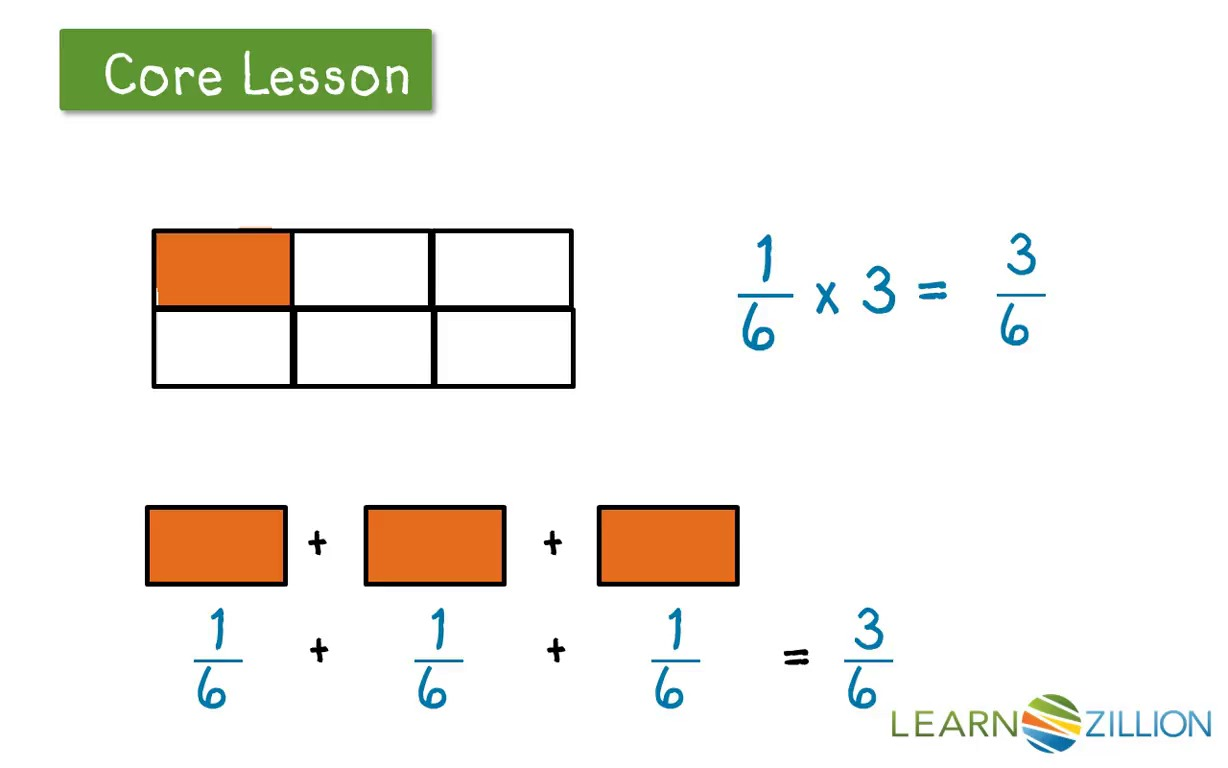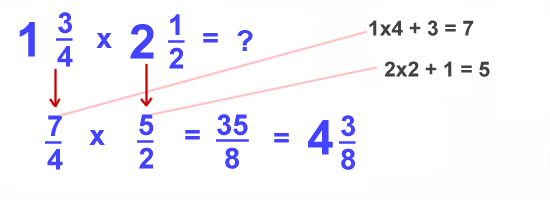### *Fractions 2 (multiply)

Multiplying fractions
1. fraction x fraction
Example:Khan Academy - fraction times fraction This is a link to an explanation of multiplying 2 fractions.
Learn Zillion - fraction times fraction This is another link to an explanation of multiplying 2 fractions.

2. fraction x whole numberLean Zillion - fraction times a whole number This is a link to an explanation of multiplying an fraction and whole number.

3. fraction x mixed number